### Derivatives, Limits, Sums and Integrals

The expressions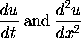are obtained in LaTeX by typing \frac{du}{dt} and \frac{d^2 u}{dx^2} respectively. The mathematical symbol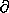is produced using \partial. Thus the Heat Equation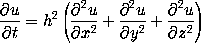is obtained in LaTeX by typing
$\frac{\partial u}{\partial t} = h^2 \left( \frac{\partial^2 u}{\partial x^2} + \frac{\partial^2 u}{\partial y^2} + \frac{\partial^2 u}{\partial z^2} \right)$


To obtain mathematical expressions such as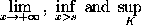in displayed equations we type \lim_{x \to +\infty}, \inf_{x > s} and \sup_K respectively. Thus to obtain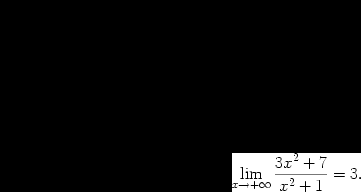(in LaTeX) we type
$\lim_{x \to +\infty} \frac{3x^2 +7x^3}{x^2 +5x^4} = 3.$


To obtain a summation sign such as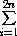we type \sum_{i=1}^{2n}. Thus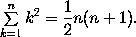is obtained by typing
$\sum_{k=1}^n k^2 = \frac{1}{2} n (n+1).$


We now discuss how to obtain integrals in mathematical documents. A typical integral is the following: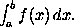This is typeset using
$\int_a^b f(x)\,dx.$

The integral signis typeset using the control sequence \int, and the limits of integration (in this case a and b are treated as a subscript and a superscript on the integral sign.

Most integrals occurring in mathematical documents begin with an integral sign and contain one or more instances of d followed by another (Latin or Greek) letter, as in dx, dy and dt. To obtain the correct appearance one should put extra space before the d, using \,. Thus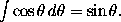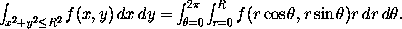and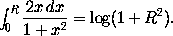are obtained by typing
$\int_0^{+\infty} x^n e^{-x} \,dx = n!.$

$\int \cos \theta \,d\theta = \sin \theta.$

$\int_{x^2 + y^2 \leq R^2} f(x,y)\,dx\,dy = \int_{\theta=0}^{2\pi} \int_{r=0}^R f(r\cos\theta,r\sin\theta) r\,dr\,d\theta.$

and
$\int_0^R \frac{2x\,dx}{1+x^2} = \log(1+R^2).$

respectively.

In some multiple integrals (i.e., integrals containing more than one integral sign) one finds that LaTeX puts too much space between the integral signs. The way to improve the appearance of of the integral is to use the control sequence \! to remove a thin strip of unwanted space. Thus, for example, the multiple integralis obtained by typing
$\int_0^1 \! \int_0^1 x^2 y^2\,dx\,dy.$

$\int_0^1 \int_0^1 x^2 y^2\,dx\,dy.$

we would have obtained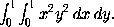A particularly noteworthy example comes when we are typesetting a multiple integral such as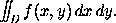Here we use \! three times to obtain suitable spacing between the integral signs. We typeset this integral using
$\int \!\!\! \int_D f(x,y)\,dx\,dy.$

$\int \int_D f(x,y)\,dx\,dy.$

we would have obtained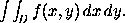The following (reasonably complicated) passage exhibits a number of the features which we have been discussing: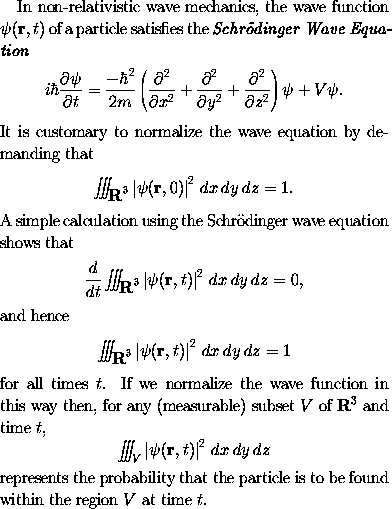One would typeset this in LaTeX by typing
In non-relativistic wave mechanics, the wave function
$\psi(\mathbf{r},t)$ of a particle satisfies the
\emph{Schr\"{o}dinger Wave Equation}
$i\hbar\frac{\partial \psi}{\partial t} = \frac{-\hbar^2}{2m} \left( \frac{\partial^2}{\partial x^2} + \frac{\partial^2}{\partial y^2} + \frac{\partial^2}{\partial z^2} \right) \psi + V \psi.$
It is customary to normalize the wave equation by
demanding that
$\int \!\!\! \int \!\!\! \int_{\textbf{R}^3} \left| \psi(\mathbf{r},0) \right|^2\,dx\,dy\,dz = 1.$
A simple calculation using the Schr\"{o}dinger wave
equation shows that
$\frac{d}{dt} \int \!\!\! \int \!\!\! \int_{\textbf{R}^3} \left| \psi(\mathbf{r},t) \right|^2\,dx\,dy\,dz = 0,$
and hence
$\int \!\!\! \int \!\!\! \int_{\textbf{R}^3} \left| \psi(\mathbf{r},t) \right|^2\,dx\,dy\,dz = 1$
for all times~$t$. If we normalize the wave function in this
way then, for any (measurable) subset~$V$ of $\textbf{R}^3$
and time~$t$,
$\int \!\!\! \int \!\!\! \int_V \left| \psi(\mathbf{r},t) \right|^2\,dx\,dy\,dz$
represents the probability that the particle is to be found
within the region~$V$ at time~$t$.